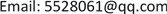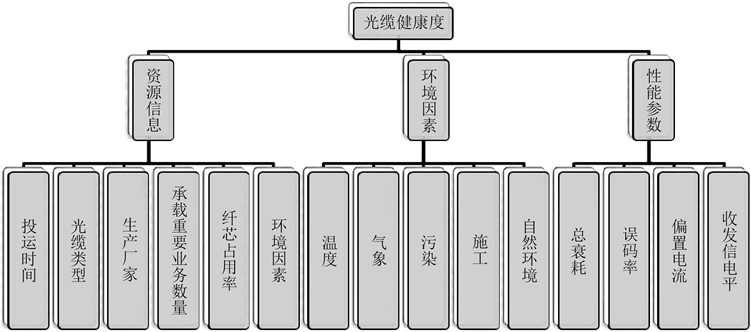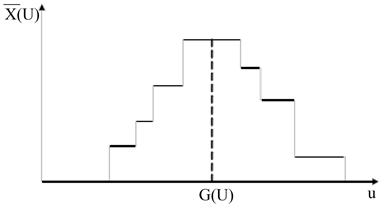﻿ 基于不确定层次分析法的电力光缆网健康度评估研究 Research on Health Assessment of Power Optical Cable Network Based on Uncertain Analytic Hierarchy Process

Smart Grid
Vol. 09  No. 02 ( 2019 ), Article ID: 29504 , 8 pages
10.12677/SG.2019.92005

Research on Health Assessment of Power Optical Cable Network Based on Uncertain Analytic Hierarchy Process

Tao Lu1, Sunli Lv1, Weiwei Miao2, Haiyang Wu2, Hao Wu3, Xinyuan Teng4

1NARI Group Corporation (State Grid Electric Power Research Institute), Nanjing Jiangsu

2State Grid Jiangsu Electric Power Co. Ltd., Nanjing Jiangsu

3State Grid Qinghai Electric Power Co. Ltd., Xining Qinghai

4Changsha University of Science & Technology, Changsha HunanReceived: Mar. 6th, 2019; accepted: Mar. 21st, 2019; published: Mar. 28th, 2019ABSTRACT

With the continuous expansion of power optical cable network scale, the network level is gradually increasing, and the network structure is becoming more and more complex, which poses new challenges to the operation and management of power communication system. As the intersection and integration point of energy and information communication technology, electric power information communication will play a greater role and assume greater responsibility. At present, the monitoring of the optical cable network still stays in response to the network problems to a large extent. The inspection management of the optical cable network is the lack of pertinence and inefficient, which affects the security and stability of the operation of communication services. Based on the uncertain analytic hierarchy process (AHP), this paper unifies the modeling of resource data, environment data and performance data indicators related to the power cable network, proposes a health evaluation model for the power cable network, and based on this model, realizes the condition-based maintenance of the optical cable network, improves the monitoring management and operation and maintenance mode of the power cable network, and provides the operation and maintenance management for the power communication. It provides better technical support.

Keywords:Analytic Hierarchy Process, Health Degree, Optical Cable Network, Condition Based Maintenance

1南瑞集团有限公司(国网电力科学研究院有限公司)，江苏 南京

2国网江苏省电力有限公司，江苏 南京

3国网青海省电力有限公司，青海 西宁

4长沙理工大学，湖南 长沙Copyright © 2019 by author(s) and Hans Publishers Inc.1. 引言

2. 电力光缆网健康评价指标分析

2.1. 指标特征分析Figure 1. Evaluation index system of fiber cable health

2.2. 指标数据获取

3. 基于不确定层次分析法的光缆健康度模型

3.1. 指标参数值的确定

3.1.1. 指标量化

3.1.2. 指标标准化

${X}^{*}=|X-a|$

3.1.3. 指标归一化

${X}^{*}=\frac{X-{X}_{\mathrm{min}}}{{X}_{\mathrm{max}}-{X}_{\mathrm{min}}}$

${X}^{*}=\frac{X}{{X}_{\mathrm{max}}}$

3.2. 指标权重算法

3.2.1. 不确定层次分析法

${w}_{j}=\frac{{\left({\prod }_{k-1}^{n}{a}_{jk}^{-}{a}_{jk}^{+}\right)}^{\frac{1}{2n}}}{{\sum }_{j=1}^{n}{\left({\prod }_{k=1}^{n}{a}_{ik}^{-}{a}_{ik}^{+}\right)}^{\frac{1}{2n}}}i,j=1,2,\cdots ,n$

1) 求出一致性检验指标CI

$\text{CI}=\frac{{\lambda }_{\mathrm{max}}}{n-1}$

2) 求出平均随机一致性指标RI，单层次判断矩阵的平均随机一致性指标RI随矩阵的维数而变动，其取值可如表1所示。Table 1. Average random consistency index value table

3) 求出相对一致性指标CR = CI/RI

CR越小，判断矩阵的一致性就越好，其极限值为0。当CR ≤ 0.1时，就可认为判断矩阵基本符合一致性条件，属于可接受的程度。如果CR > 0.1，一般认为该判断矩阵不可接受，需要重新分析赋值，直到检验通过为止。

3.2.2. 权重修正算法

1) 集值统计Figure 2. Value probability distribution graph

$\stackrel{¯}{X}\left(U\right)=\frac{1}{n}\underset{k=1}{\overset{n}{\sum }}{\omega }_{k}{x}_{\left[{u}_{1}^{\left(k\right)},{u}_{2}^{\left(k\right)}\right]}\left(u\right)=\frac{1}{n}\underset{k=1}{\overset{n}{\sum }}{\omega }_{k}{p}_{k},0\le \stackrel{¯}{X}\left(U\right)\le 1$

${p}_{k}={x}_{\left[{u}_{1}^{\left(k\right)},{u}_{2}^{\left(k\right)}\right]}\left(u\right)=0$

2) 重心决策

$G\left(U\right)=\frac{{\int }_{A}\stackrel{¯}{X}\left(U\right)u\text{d}u}{{\int }_{A}\stackrel{¯}{X}\left(U\right)\text{d}u},其中\underset{A}{\int }\stackrel{¯}{X}\left(U\right)\text{d}u\ne 0$

$\underset{{u}_{\mathrm{min}}}{\overset{{u}_{\mathrm{max}}}{\int }}\stackrel{¯}{X}\left(U\right)\text{d}u=\underset{k=1}{\overset{n}{\sum }}{\omega }_{k}\left[{u}_{1}^{\left(k\right)}-{u}_{2}^{\left(k\right)}\right]$

$\underset{{u}_{\mathrm{min}}}{\overset{{u}_{\mathrm{max}}}{\int }}\stackrel{¯}{X}\left(U\right)u\text{d}u=\frac{1}{2}\underset{k=1}{\overset{n}{\sum }}{\omega }_{k}\left[{\left({u}_{1}^{\left(k\right)}\right)}^{2}-{\left({u}_{2}^{\left(k\right)}\right)}^{2}\right]$

$G\left(U\right)=\frac{{\int }_{A}\stackrel{¯}{X}\left(U\right)u\text{d}u}{{\int }_{A}\stackrel{¯}{X}\left(U\right)\text{d}u}=\frac{{\int }_{{u}_{\mathrm{min}}}^{{u}_{\mathrm{max}}}\stackrel{¯}{X}\left(U\right)u\text{d}u}{{\int }_{{u}_{\mathrm{min}}}^{{u}_{\mathrm{max}}}\stackrel{¯}{X}\left(U\right)\text{d}u}=\frac{1}{2}\frac{{\sum }_{k=1}^{n}{\omega }_{k}\left[{\left({u}_{1}^{\left(k\right)}\right)}^{2}-{\left({u}_{2}^{\left(k\right)}\right)}^{2}\right]}{{\sum }_{k=1}^{n}{\omega }_{k}\left[{u}_{1}^{\left(k\right)}-{u}_{2}^{\left(k\right)}\right]}$

$\stackrel{¯}{X}\left(U\right)=\left\{\begin{array}{cc}\begin{array}{l}1\\ 0\end{array}& \begin{array}{l}u=e\\ u\ne e\end{array}\end{array}$

$G\left(U\right)=e$

4. 实例分析

4.1. 权重计算

4.2. 状态检修

5. 结论

Research on Health Assessment of Power Optical Cable Network Based on Uncertain Analytic Hierarchy Process[J]. 智能电网, 2019, 09(02): 41-48. https://doi.org/10.12677/SG.2019.92005

1. 1. 施健, 缪巍巍, 吴海洋. 基于LS-SVM的电力通信网性能劣化评估与预测模型研究[J]. 计算机与数字工程, 2016, 44(4).

2. 2. 聂勇. 变电站设备巡视的要因分析[J]. 科技资讯, 2014, 12(14): 115.

3. 3. 陈珊珊. 变电站故障巡视检查管理的关键点[J]. 科技资讯, 2014, 12(6): 151.

4. 4. Basole, R.C. (2014) Visual Business Ecosystem Intelligence: Lessons from the Field. IEEE Computer Graphics & Applications, 34, 26-34.

5. 5. 范李平, 姚迪. 基于智能化变电站的缺陷维护管理模式探讨[J]. 电气开关, 2015, 53(6): 68-72.

6. 6. 白丽锐, 杨友朋, 王春新. 数据标准化在电力物联网综合业务平台的应用[J]. 物联网技术, 2013(8): 49-53.

7. 7. 魏毅强. 不确定型AHP中判断矩阵的一致性概念及评分权重[J]. 系统工程理论与实践, 1994, 14(4): 16-22.

8. 8. 万明杰, 吕欣欣, 张汉锋. 集值统计法及其应用研究[J]. 弹箭与制导学报, 2005, 25(3): 395-396.

9. 9. 彭泽武, 李伟清, 林强, 刘文彬. 电网企业信息系统运行方式模型研究[J]. 电力信息化, 2013, 11(6): 12-17.

10. 10. 陈晰, 李娜, 吴帆, 李祥珍. 电力物联网中的分层聚合技术研究[J]. 电力系统通信, 2011, 32(12): 73-77.